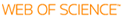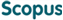### BROWSE

#### Related ResearcherRyu, Dongsu
Astrophysics Lab
Research Interests
• Shock waves and turbulence in clusters of galaxies
• Origin and evolution of magnetic fields and cosmic rays in the universe
• Plasma astrophysical phenomena in the large-scale structure of the universe

FORMATION OF PROTO-GLOBULAR CLUSTER CLOUDS BY THERMAL INSTABILITY

Cited 0 times inCited 0 times inTitle
FORMATION OF PROTO-GLOBULAR CLUSTER CLOUDS BY THERMAL INSTABILITY
Author
Keywords
galaxy; globular clusters; general; hydrodynamics; instabilities
Issue Date
2000-10
Publisher
KOREAN ASTRONOMICAL SOCIETY
Citation
JOURNAL OF THE KOREAN ASTRONOMICAL SOCIETY, v.333, no.2, pp.111 - 121
Abstract
Many models of globular cluster formation assume the presence of cold dense clouds in early universe. Here we re-examine the Fall & Rees (1985) model for formation of proto-globular cluster clouds (PGCCs) via thermal instabilities in a protogalactic halo. We first argue, based on the previous study of two-dimensional numerical simulations of thermally unstable clouds in a stratified halo of galaxy clusters by Real et al. (1991), that under the protogalactic environments only nonlinear (${\delta}{\ge}1$ 수식 이미지) density inhomogeneities can condense into PGCCs without being disrupted by the buoyancy-driven dynamical instabilities. We then carry out numerical simulations of the collapse of overdense douds in one-dimensional spherical geometry, including self-gravity and radiative cooling down to T = $10^4$ 수식 이미지 K. Since imprinting of Jeans mass at $10^4$ 수식 이미지 K is essential to this model, here we focus on the cases where external UV background radiation prevents the formation of $H_2$ 수식 이미지 molecules and so prevent the cloud from cooling below $10^4$ 수식 이미지 K. The quantitative results from these simulations can be summarized as follows: 1) Perturbations smaller than $M_{min}\~(10^{5.6}\;M{\bigodot})(nh/0.05cm^{-3})^{-2}$ 수식 이미지 cool isobarically, where nh is the unperturbed halo density, while perturbations larger than $M_{min}\~(10^8\;M{\bigodot})(nh/0.05cm^{-3})^{-2}$ 수식 이미지 cool isochorically and thermal instabilities do not operate. On the other hand, intermediate size perturbations ($M_{min} < M_{pgcc} < M_{max}$) are compressed supersonically, accompanied by strong accretion shocks. 2) For supersonically collapsing clouds, the density compression factor after they cool to $T_c = 10^4$ 수식 이미지 K range $10^{2.5} - 10^6$ 수식 이미지, while the isobaric compression factor is only $10^{2.5}$ 수식 이미지. 3) Isobarically collapsed clouds ($M < M_{min}$) are too small to be gravitationally bound. For supersonically collapsing clouds, however, the Jeans mass can be reduced to as small as $10^{5.5}\;M_{\bigodot}(nh/0.05cm^{-3})^{-1/2}$ 수식 이미지 at the maximum compression owing to the increased density compression. 4) The density profile of simulated PGCCs can be approximated by a constant core with a halo of $p{\infty} r^{-2}$ 수식 이미지 rather than a singular isothermal sphere.
URI
ISSN
1225-4614
Appears in Collections:
PHY_Journal Papers
Files in This Item: